# How to do Regression Analysis In Excel

Regression is an Analysis Tool, which we use for analyzing large amounts of data and making forecasts and predictions in Microsoft Excel.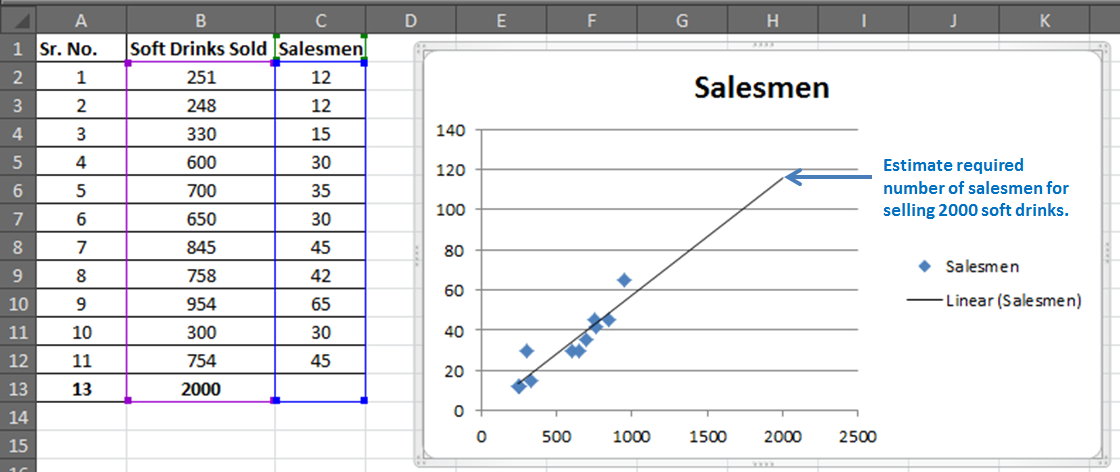Want to predict the future? No, we are not going to learn astrology. We are into numbers and we will learn regression analysis in Excel today.

To predict future estimates, we will study:

Let’s do it...

Scenario:

Let’s assume you sell soft drinks. How cool will it be if you can predict:

• How many soft drinks will be sold next year based on previous year's data?
• Which fields need to be focused?
• And how can you increase your sales by changing your strategy?

It will be profitably awesome. Right?… I know. So let’s get started.

You have 11 records of salesmen and soft drinks sold.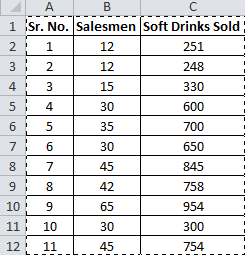Now based on this data you want to predict the number of salesmen required to achieve 2000 sales of soft drinks.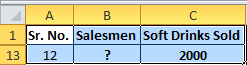The regression equation is a tool to make such close estimates. To do so, we need to know Regression first.

## REGRESSION ANALYSIS USING EXCEL FUNCTIONS (MANUAL REGRESSION FINDING)

This part will make you understand regression better than just telling excel regression procedure.

Introduction:

Simple Linear Regression:

The study of the relationship between two variables is called Simple Linear Regression. Where one variable depends on the other independent variable. The dependent variable is often called by names such as Driven, Response, and Target variable. And the independent variable is often pronounced as a Driving, Predictor or simply Independent variable. These names clearly describe them.Now let’s compare this with your scenario. You want to know the number of salesmen required to achieve 2000 sales. So here, the dependent variable is the number of salesmen and the independent variable is sold soft drinks.

The independent variable is mostly denoted as x and dependent variable as y.

In our case, soft drinks are sold x and the number of salesmen is y.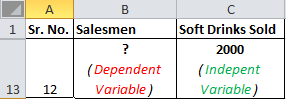If we want to know how many soft drinks will be sold if we appoint 200 salesmen, then the scenario will be vice-versa.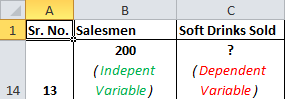Moving On.

The “Simple” Math of Linear Regression Equation:

Well, it's not simple. But Excel made it simple to do.

We need to predict the required number of salesmen for all 11 cases to get the 12th closest prediction.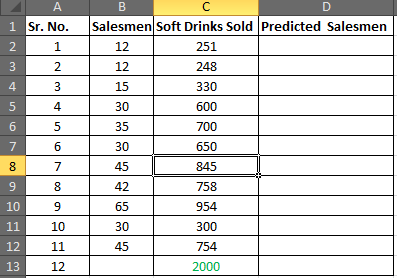Let’s say:

Soft Drink Sold is x

The number of Salesmen is y

The predicted y (number of salesmen) also called Regression Equation,  would be

 x*Slope+Intercept   (relax, I’ve got it covered)

Now you must be wondering where the stat will you get the slope and intercept. Don’t worry, excel has functions for them. You do not need to learn how to find the slope and intercept it manually.

If you want, I will prepare a separate tutorial for that. Let me know in the comments section. These are some important data analytics tools.

Now let’s step into our calculation:

Step1: Prepare this small table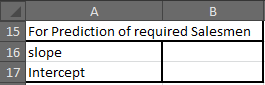Step 2: Find the slope of the regression line

Excel Function for slopes is

 =SLOPE(known_y’s,known_x’s)

Your known_y’s are in range B2:B12 and known_x’s are in range C2:C12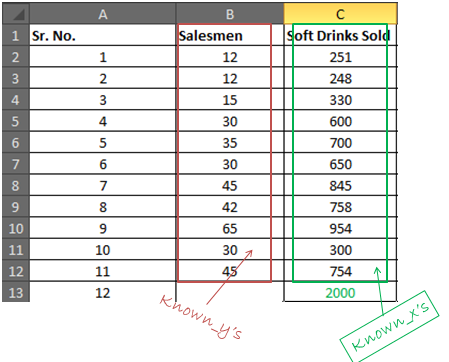In cell B16, write the formula below

 =SLOPE(B2:B12, C2:C12)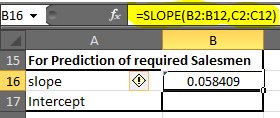(Note: Slope is also called coefficient of x in the regression equation)

You will get 0.058409. Round up to 2 decimal digits and you will get 0.06.

Step 3: Find the Intercept of Regression Line

Excel function for the intercept is

 =INTERCEPT(known_y’s, known_x’s)

We know what our known x’s and y’s

In cell B17, write down this formula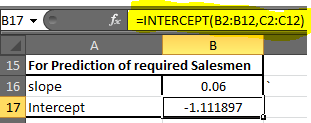=INTERCEPT(B2:B12, C2:C12)

You will get a value of -1.1118969. Roundup to 2 decimal digits. You will get -1.11.

Our Linear Regression Equation is = x*0.06 + (-1.11). Now we can predict possible y depending on the target x easily.

Step 4: In D2 write the formula below

 =C2*\$B\$16+\$B\$17    (Regression Equation)

You will get a value of 13.55.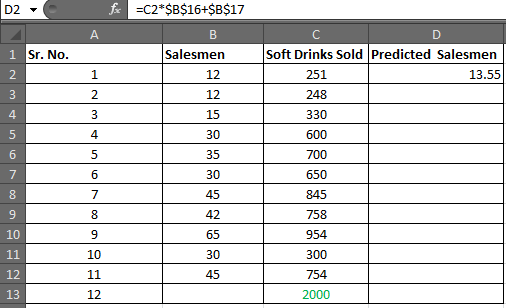Select D2 to D13 and press CTRL+D to fill down the formula in the range D2:D13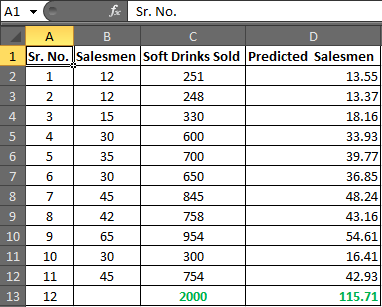In cell D13 you have your required number of salesmen.

 Hence, to achieve the target of 2000 Soft Drink Sales, you need an estimate of 115.71 salesmen or say 116 since it is illegal to cut humans into pieces.

Now using this you can easily conduct What-If analysis in excel. Just change the number of sales and it will show you many salesmen will it take to get that sales target achieved.

Play around it to find out:

How much workforce do you need to increase sales?

How many sales will increase if you increase your salesmen?

## Make Your Estimate More Reliable:

Now you know that you need 116 salesmen to get 2000 sales done.

In analytics, nothing is just said and believed. You must give a percentage of reliability on your estimate. It is like giving a certificate of your equation.Correlation Coefficient Formula:

The next thing you will be asked is how much these two variables are related. In static terms, you need to tell the coefficient of correlation.

Excel function for correlation is

 =CORREL(array1, array2)

In your case, known_x’s and Know_y’s are array1 and array2 irrespectively.

In B18 enter this formula

 =CORREL((B2:B12, C2:C12)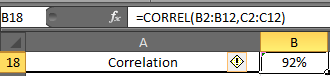You will have 0.919090. Formate cell B2 into the percentage. Now have 92% of correlation.

Now, what this 92% means. It means, there 92% of chances of sales increase if you increase the number of salesmen and 92% of sales decrease if you decrease the number of salesmen. It is called Positive Correlation Coefficient.

R Squire (R^2) :

R Squire value tells you, by what percentage your regression equation is not a fluke. How much it is accurate by the data provided.

The Excel function for R squire is RSQ.

 RSQ(known_y’s, Known_x’s)

In our case, we will get R squire value in cell B19.

In B19 enter this formula

 =RSQ(B2:B12, C2:C12)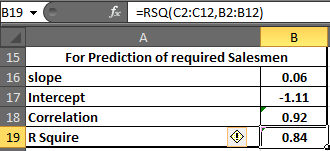So we have 84% of r Square value. Which is a very good explanation of our regression. It says that 84% of our data is just not by chance. Y (number of salesmen) is very much dependent on X (sales of soft drinks).

There are many other tests we can do on this data to ensure our regression. But manually it will be a complex and lengthy procedure. That is why excel provides Analysis Toolpak. Using this tool we can do this regression analysis in seconds.

## REGRESSION IN EXCEL USING EXCEL’S ANALYSIS TOOLPAK ADD-IN

If you already know what regression equations are, and you just want your results quickly then this part is for you. But if you want to understand regression equations easily then scroll up to REGRESSION ANALYSIS USING EXCEL FUNCTIONS (MANUAL REGRESSION FINDING).

Excel provides a whole bunch of tools for analysis in its Analysis Toolpak. By default, it is not available in the Data tab. You need to add it. So let’s add it first.

## Adding Analysis Toolpak to Excel 2016

If you don’t know where is data analysis in excel follow these steps

Step 1: Go to Excel Options: File? Options? Add-Ins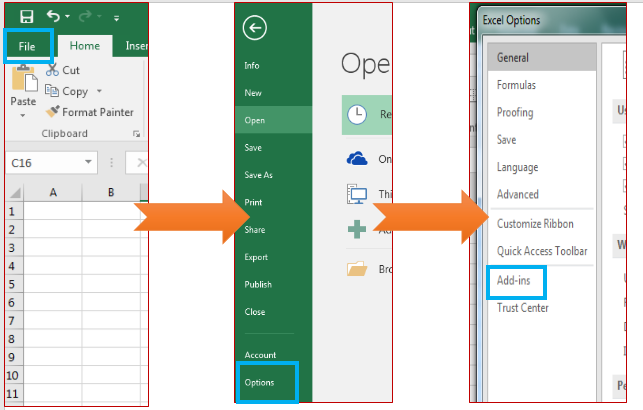Step 2: Click on Add-Ins. You will see a list of available add-Ins.

Select Analysis ToolPak and at the bottom of the window, find manage. In manage select Excel Add-Ins and Click on GO.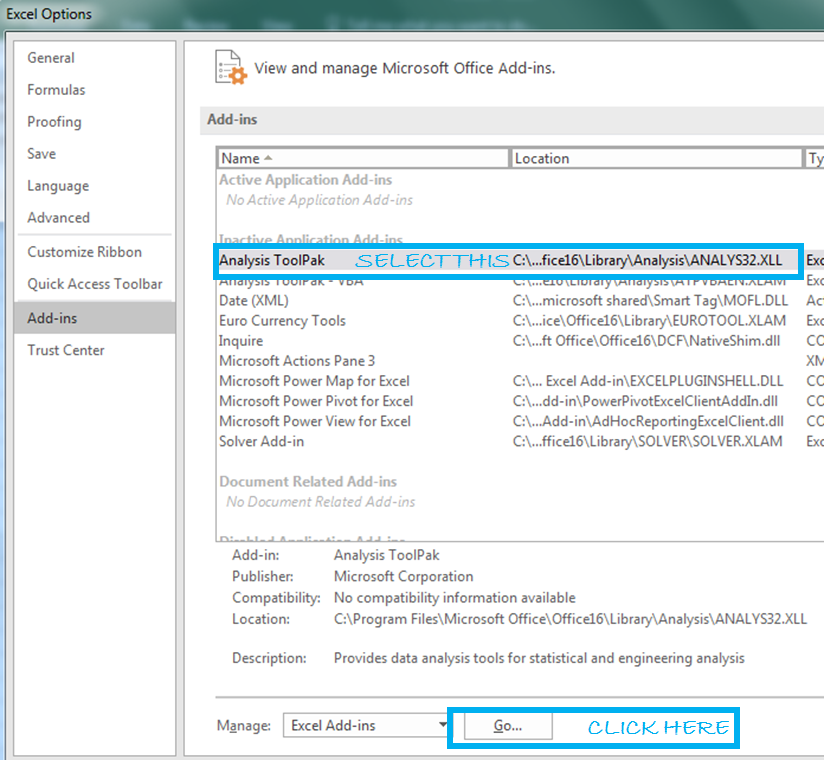Add-ins window will open. Here, select Analysis ToolPak. Then click the ok button.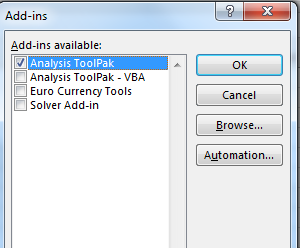Now you can access all functions of data analysis ToolPak from Data Tab.

## Using Analysis ToolPak for Regression

Step 1: Go to the Data tab, Locate Data Analysis. Then click on it.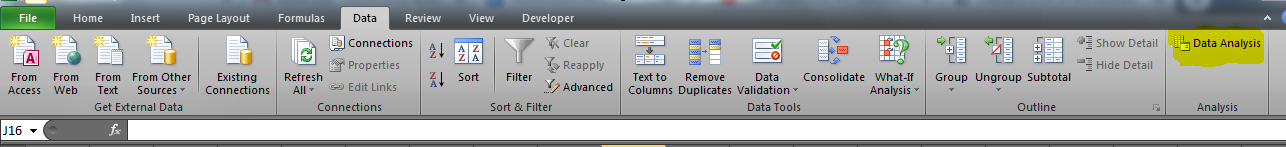A dialogue box will pop up.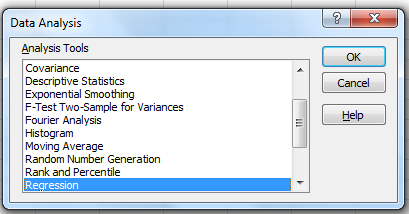Step 2: Find ‘Regression’ in Analysis Tools list and hit the OK button.

The regression input window will pop up. You will see a number of available input options. But for now, we will just concentrate on Y Range and X Range, leaving everything else to default.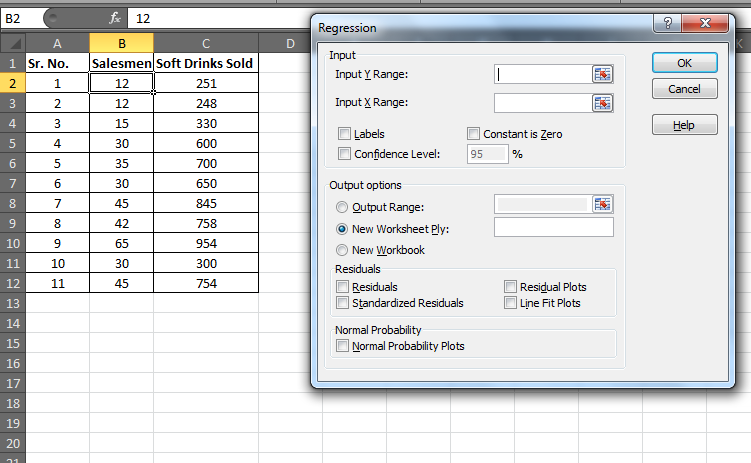Step 4: Provide Inputs: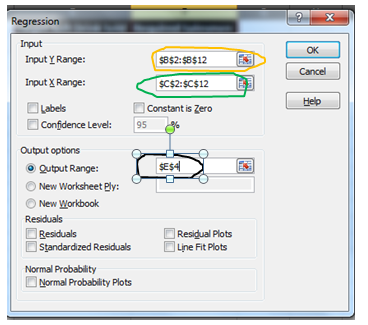No. of Salesmen is Y

Sales of soft drinks are X

Hence

• Y Range= B2:B11

And

•  X Range = C2:C11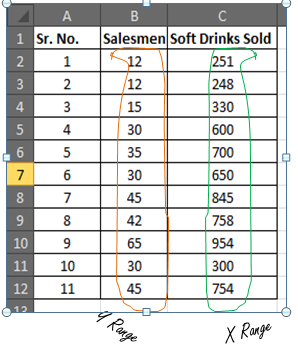For the output range, I have selected E4 on the same sheet. You may select a new worksheet to get results on a new worksheet in the same workbook or a complete new workbook. When you are done with your inputs, hit the OK button.

Results:

You will be served with a variety of information from your data. Don’t get overwhelmed. You don’t need to consume all the dishes.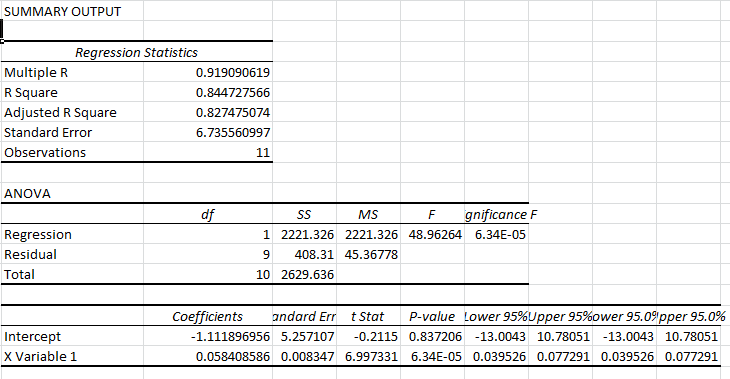We will only deal with those results which will help us to estimate the required number of salesmen

Step 5: We know the regression equation for estimation of y, that is

x*Slope+Intercept

We just need to locate Slope and Intercept in results.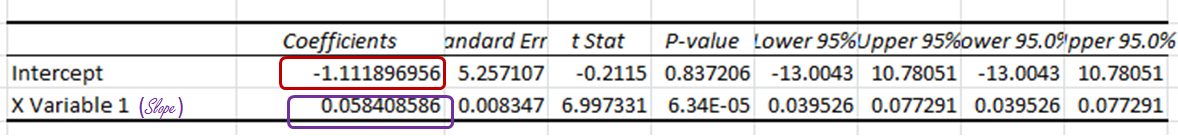And here they are.

The intercept Coefficient is clearly mentioned.

The slope is written as ‘X Variable 1’, some times also mentioned as the coefficient of X. Round up them and we will get -1.11 as Intercept and 0.06 as Slope.

Step 6: From results, we can drive the Regression equation. And that would be

=x*(0.06) + (-1.11)

Prepare this table in excel.For now, x is 2000, which is in cell E2.

In Cell F2 enter this formula

You will get a result of 115.7052757.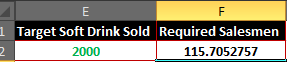Rounding it up will give us 116 of Required Salesmen.

So we have learned how to form the regression equation manually and using Analysis ToolPak. How can you use this equation to estimate future stats?

Now let’s understand the regression output given by Analysis Toolpak.

## Understanding the Regression Output:

There is no benefit, if you do regression analysis using analysis tool pack in excel and can’t interpret its meaning.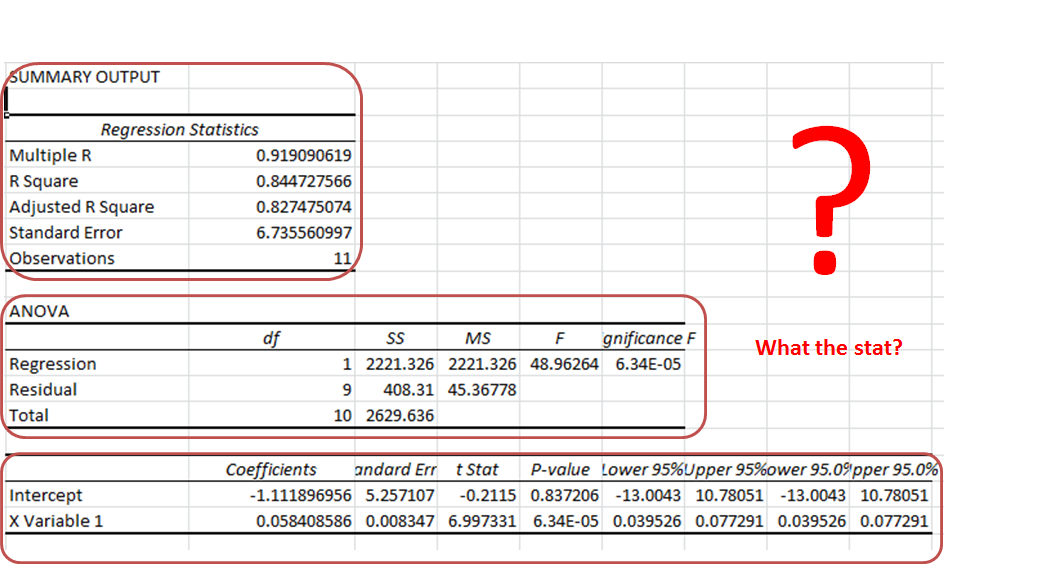Summary Section:

As the name suggests, it is a summary of the data.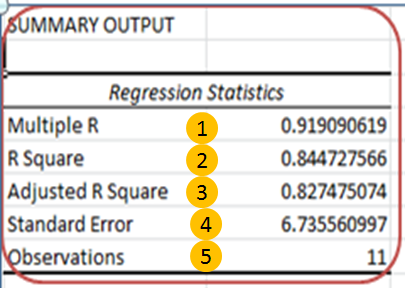1. Multiple R: It tells how fit the regression equation is to the data. It is also called the correlation coefficient.

In our case, it is 0.919090619 or 0.92 (roundup). This means that there is a 92% chance of an increase in sales if we increase our salesmen count.

1. R Square: It tells the reliability of found regression. It tells us how many observations are part of our line of regression. In our case, it is 0.844727566 or 0.85. It means that our regression is fit by 85%.
2. Adjusted R Square: Theadjusted square is just a more testified version of R square. Mainly useful in Multiple Regression Analysis.
3. Standard Error: While R. Squire tells you how many data points fall near the regression line, the standard error tells you how far a data point can go from the regression line.

In our case, it is 6.74.

1. Observation: This is simply the number of observations, which is 11 in our example.

Anova Section:

This section is hardly used in linear regression.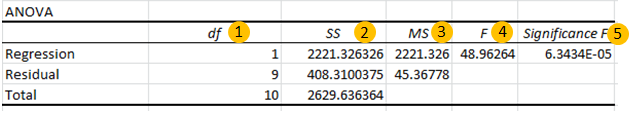1. df. It is a degree of freedom. It is used when calculating regression manually.
2. SS. Sum of squares. It is just a sum of squares of variances. Used to find R squire values.
3. MS. This means squared value.
4. And 5. F and Significance of F. If the significance of F (p-value of the slope) is less than the F test than you can discard the null hypothesis and prove your hypothesis. In simple language, you can conclude that there is some effect of x on y when changed.

In our case, F is 48.96264 and Significance of F is 0.000063. It means our regression fits the data.

Regression Section: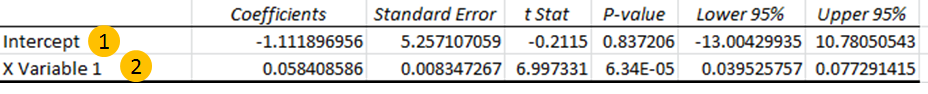In this section, we have the two most important values for our regression equation.

1. Intercept: We have an intercept here that tells where x-intercepts on Y. This is an important part of the regression equation. It is -1.11 in our case.
2. X variable 1 (Slope). Also called the coefficient of x. It defines the tangent of the regression line.

## REGRESSION CHART IN EXCEL

In excel, it is easy to plot a regression chart. Just follow these steps. To add Regression Chart in Excel 2016, 2013, and 2010 follow these simple steps.

Step 1. Have your known x’s in the first column and know y’s in the second.

In our case, we know Known_ x’s are Soft Drinks Sold. And known_y’s are Salesmen.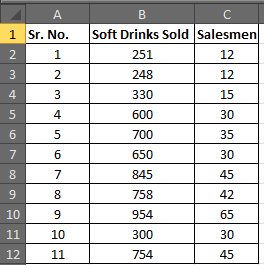Step 2. Select your known x’s and y’s range.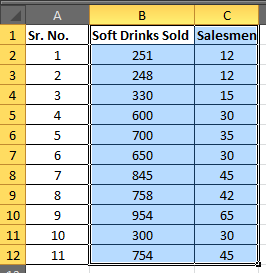Step 3: Go to the Insert tab and click on the scatter chart.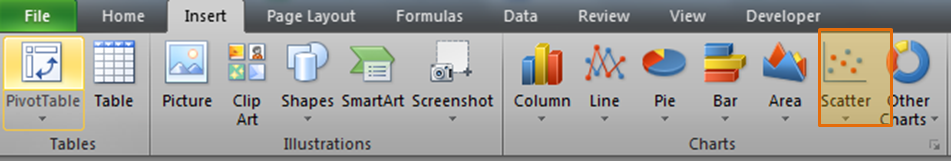You will have a chart that looks like this.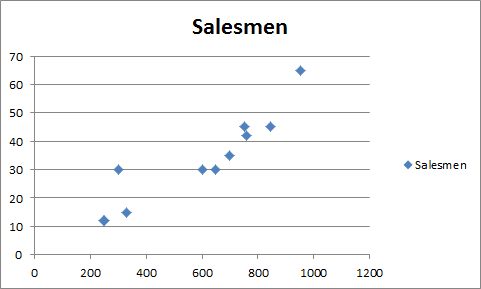Step 4. Add the trend line: Goto layout and locate the trendline option in the analysis section.

Under the Trendline option, click on Linear Trendline.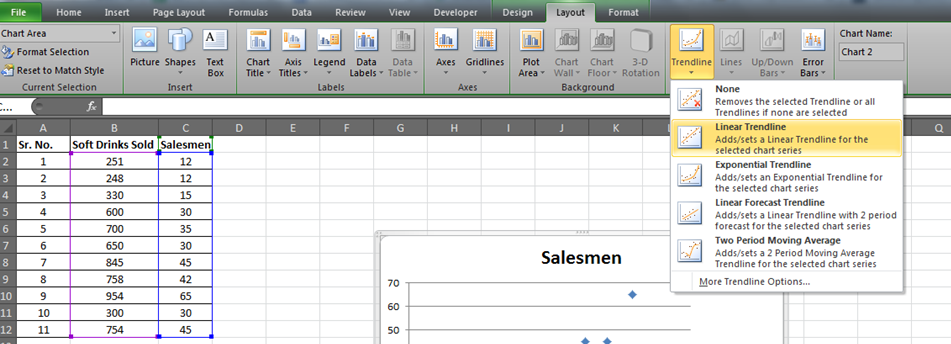You will have your graph looking like this.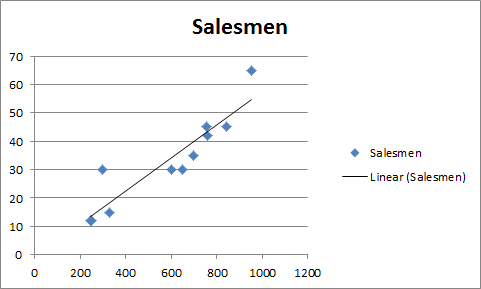Now if you add the data below and extend the selected data. You will see a change in your graph.

For our example, we added 2000 to the Soft Drink Sold and left the Salesmen blank. And when we extend the range of the graph, this is what we will have.

It will give the required number of salesmen for doing 2000 sales of soft drinks in graphical form. Which is slightly below 120 in the graph. And from our regression equation, we know it is 116.In this article, I tried to cover everything under Excel Regression Analysis. I explained regression in excel 2016. Regression in excel 2010 and excel 2013 is same as in excel 2016.

For any further query on this topic, use the comments section. Ask a question, give an opinion or just mention my grammatical mistakes. Everything is welcome. Just don’t hesitate to use the comment section.

Related Data:
How to Use STDEV Function in Excel

How to Create Standard Deviation Graph

Descriptive Statistics in Microsoft Excel 2016

How to Use Excel NORMDIST Function

How to use the Pareto Chart and Analysis

Popular Articles:

50 Excel Shortcut to Increase Your Productivity

How to use the VLOOKUP Function in Excel

How to use the COUNTIF function in Excel 2016

How to use the SUMIF Function in Excel

## Users are saying about us...

1.nice and interesting blog

2.Testing

•Thanks

Terms and Conditions of use

The applications/code on this site are distributed as is and without warranties or liability. In no event shall the owner of the copyrights, or the authors of the applications/code be liable for any loss of profit, any problems or any damage resulting from the use or evaluation of the applications/code.